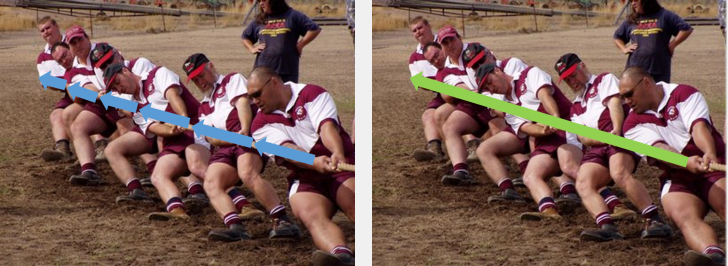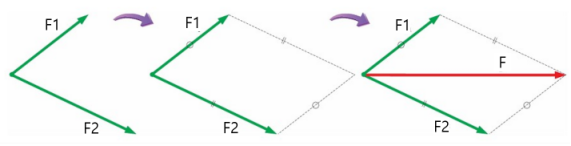Three elements of power

When a force is applied to an object, the movement of the object depends on the ‘magnitude’ of the force, the ‘direction’ of the force, and the ‘point of action’ of the force. This is called the third element of force. Therefore, when displaying force, you must indicate the size, direction, and point of action.Let’s think of sliding a book on a desk. If the pushing position is midway, the book moves in the pushing direction. When the pushing position is at the end of the book, the book is pushed and rotated. That is, depending on the point where the force acts, the object can only translate or rotate.

Net powers

It can be represented as a force by combining several forces, called net force.If you combine all the forces of the tug-of-war you can be represented by a single big arrow.

Finding the resultant force (diagonal method)

Draw two forces in an arrow, then draw a parallelogram of arrows. At this point, the diagonal arrow from the point of action of the parallelogram becomes the net forces. The length of the diagonal line indicates the magnitude of the net forces, and the diagonal line indicates the direction of the net forces.The larger the angle between the two forces, the smaller the size of the net force.
When the angle between the two forces is 0 °, the two forces act in the same direction, so the magnitude of the net force is maximum.If the angle between the two forces is 120 ° and the magnitudes of the two forces are the same, the magnitude of the net force is equal to the magnitude of the individual force before the addition. When the angle between the two forces is 180 °, the net force is minimized because both forces act in opposite directions.